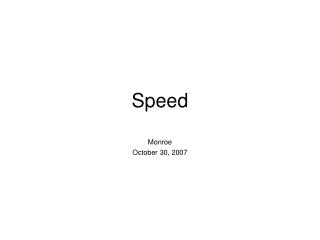DownloadDownload PresentationSpeed

# Speed

Download Presentation## Speed

- - - - - - - - - - - - - - - - - - - - - - - - - - - E N D - - - - - - - - - - - - - - - - - - - - - - - - - - -
##### Presentation Transcript

1. Speed Monroe October 30, 2007

2. Speed ~ distance divided by time Continents move in centimeters per year Airplanes in kilometers per hour American cars in miles per hour Comets in miles per second Bullets in feet per second These are all measurements of speed

3. Average speed is over a longer period of time. If Sam Swiftfoot can run 10 miles in 73 minutes, what is his average speed?

4. Average speed is over a longer period of time. If Sam Swiftfoot can run 10 miles in 73 minutes, what is his average speed? 10 miles 60 minutes 8.22 miles ----------- x -------------- = --------------- 73 minutes 1 hour hour

5. Instantaneous Speed ~ is the speed during an instant of time. Look at your speedometer for a good idea. There really is no such number as instantaneous speed, however, because one can always reduce time until it reaches zero, at which time the number becomes undefined. One cannot divide by zero.

6. Velocity ~ is the speed in GIVEN DIRECTION. This implies displacement instead of distance.

7. Velocity ~ is the speed in GIVEN DIRECTION. This implies displacement instead of distance. Distance ~ is how far you move, along the face of the Earth, compared to a reference point.

8. Velocity ~ is the speed in GIVEN DIRECTION. This implies displacement instead of distance. Distance ~ is how far you move, along the face of the Earth, compared to a reference point. Displacement ~ is the distance between the starting point and the ending point of a movement.

9. A horse took 15 seconds to run around an oval track, that was 1/4 mile in length. What is the total distance that he ran?

10. A horse took 15 seconds to run around an oval track, that was 1/4 mile in length. What is his total displacement?

11. A horse took 15 seconds to run around an oval track, that was 1/4 mile in length. What was his average speed?

12. A horse took 15 seconds to run around an oval track, that was 1/4 mile in length. What was his average volocity?